{{announcement.body}}
{{announcement.title}}

# Power of Arrays Module in DataWeave

DZone 's Guide to

# Power of Arrays Module in DataWeave

### Let's look at the power of the arrays module in DataWeave.

· Integration Zone ·
Free Resource

Comment (0)

Save
{{ articles.views | formatCount}} Views

We are well aware that DataWeave plays an important role in developing integration applications using MuleSoft. It also contains a lot of modules that enhance its power. Out of all of these modules, we have the Arrays module (dw::core::Arrays), which helps us utilize arrays in our integration application efficiently.

 countBy indexWhere some divideBy join splitAt drop leftJoin splitWhere dropWhile outerJoin sumBy every partition take indexOf slice takeWhile

Let us now go into the details of each function.

1. countBy

With the help of this function, we can find out the number of elements in an array as per a Boolean expression, it will look for the elements in a list (array) that match the results of the function.

Parameters

• Name of Array
• General Expression or Lambda Expression returning a Boolean value.

Syntax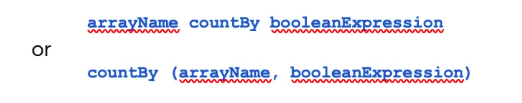Example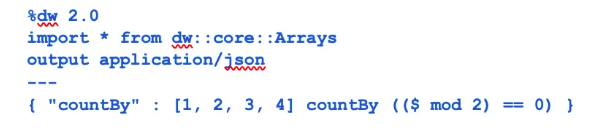Output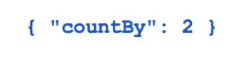1. divideBy

The divideBy function can be used to break up a list (array) into sub-lists (sub-arrays) that contain the specified number of elements. When there are fewer elements in the input array than the specified number, the function fills the sub-array with those elements. When there are more elements, the function fills as many sub-arrays needed with the extra elements.

Parameters

• Name of Array
• Number of elements in the sub-lists

Example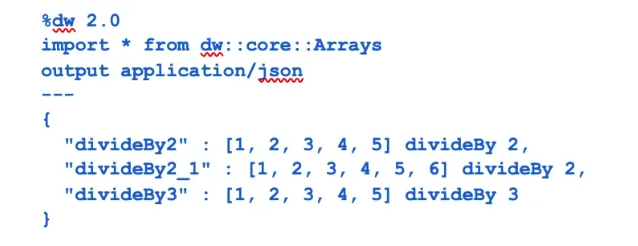Output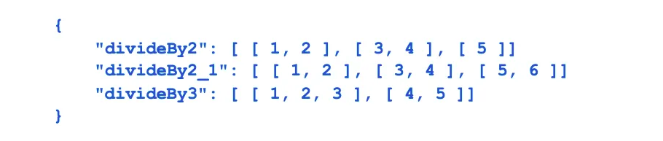1. drop

Drop function will drop first n elements. It returns the original array when n <= 0 and an empty array when n > sizeOf(array).

Parameters:

• Array Name
• Number of elements to drop

Example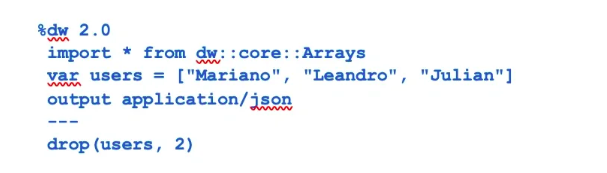Output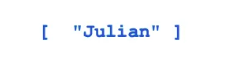1. dropWhile

Similar to the previous drop function but it will drop as per a Boolean expression.

Parameters

• Array Name
• Boolean Expression

Example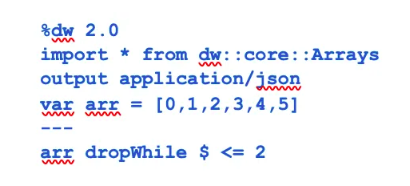Output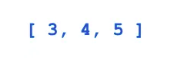1. every

Returns true if every element in the array matches the boolean expression. The function stops iterating after the first negative evaluation of an element in the array.

Parameters

• Array Name
• Boolean Expression

Example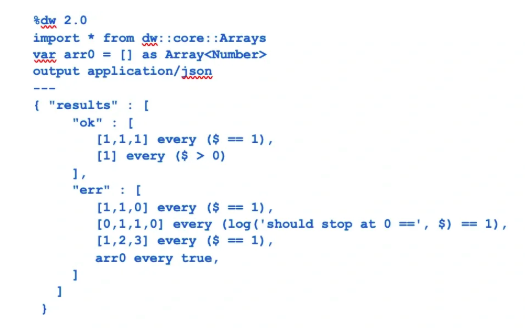Output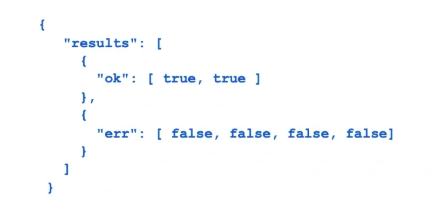1. indexOf

indexOf function returns the index of the first occurrence of an element within the array.

Parameters

• Array Name
• Any Value

Example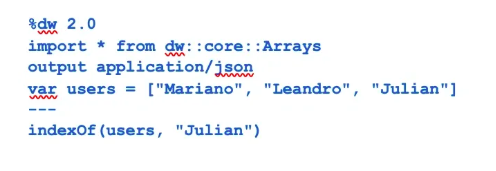Output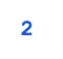1. indexWhere

indexWhere can be used to return the index of the first occurrence of an element that matches a condition within the array.

Parameters

• Array Name
• Boolean Expression

Example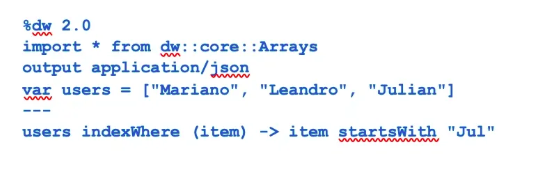Output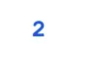1. join

Joins two Arrays of Objects by an ID criterion. It will return an Array of Objects containing only the objects for which the ID from the left group can be matched with an ID from the right group.

Parameters

• Left Array of Objects
• Right Array of Objects
• Left Criteria to extract the key column for the left collection.
• Right Criteria to extract the key column for the right collection.

Example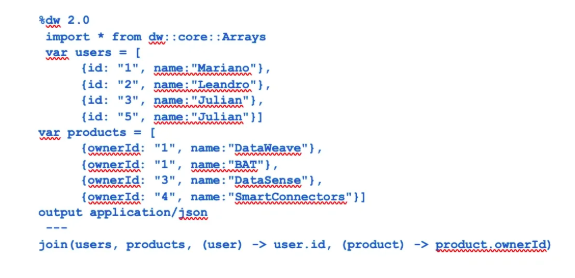Output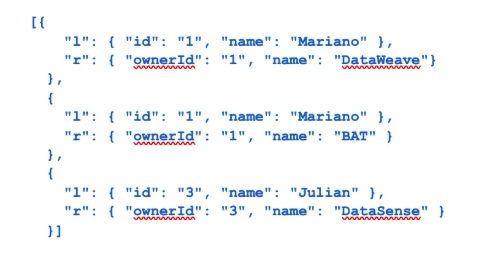1. leftJoin

Joins two Arrays of Objects by a given ID criterion. It will return an Array of all the left items merged by ID with the right items if any exists.

Parameters

• Left Array of Objects
• Right Array of Objects
• Left Criteria to extract the key column for the left collection.
• Right Criteria to extract the key column for the right collection.

Example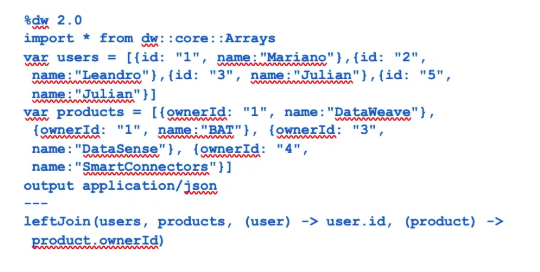Output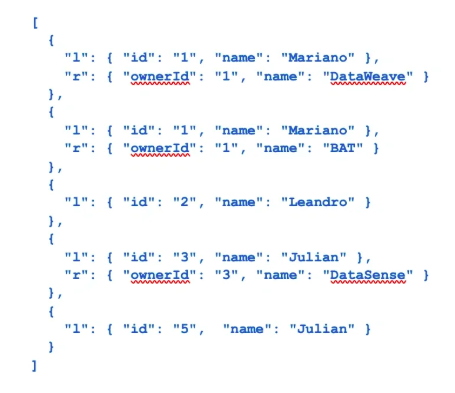1. outerJoin

Joins two Arrays of Objects by a given ID criterion. It will return an Array with all the left items merged by ID with the left items if any exists, and the right items that are not present in the left.

Parameters

• Left Array of Objects
• Right Array of Objects
• Left Criteria to extract the key column for the left collection.
• Right Criteria to extract the key column for the right collection.

Example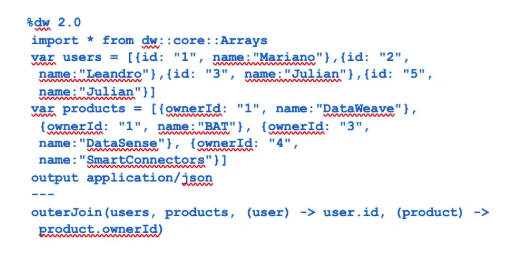Output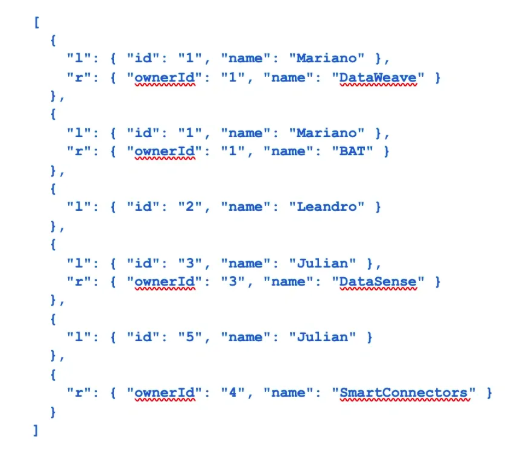1. partition

Separates the array into the elements that satisfy the condition and those that don't.

Parameters

• Array Name.
• Boolean/Lambda Expression returning a boolean value.

Example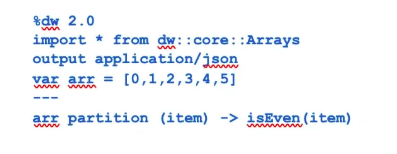Output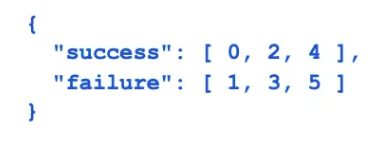1. slice

Selects the interval of elements that satisfy the condition: from <= indexOf(array) < until

Parameters

• An Array
• Starting Index of the slice
• End Index of the slice

Example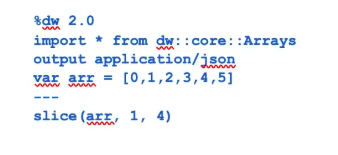Output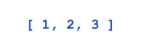1. some

Returns true if an element in the array matches the specified condition. The function stops iterating after the first match to an element in the array.

Parameters

• The input array.
• A condition (or expression) used to match elements in the array.

Example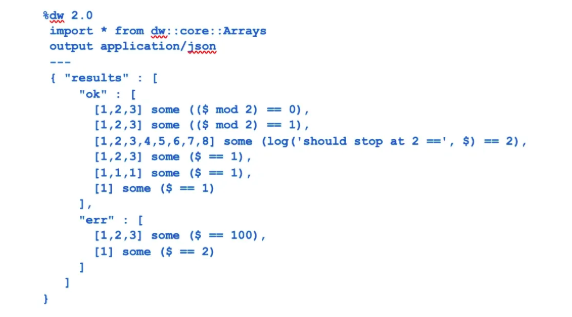Output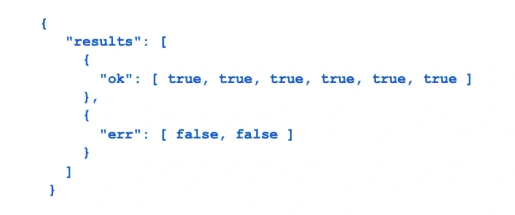1. splitAt

Splits an array into two at a given position.

Parameters

• The Array of elements.
• The index to split at.

ExampleOutput1. splitWhere

Splits an array into two at the first position where the condition is met.

Parameters

• The Array of elements to split.
• The condition (or expression) used to match an element in the array.

Example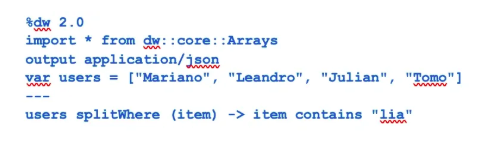Output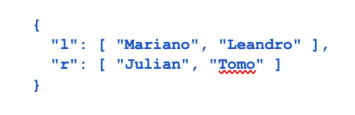1. sumBy

Returns the sum of the values of the elements in an array.

Parameters

• The input array.
• A DataWeave selector that selects the values of the numbers in the input array.

Example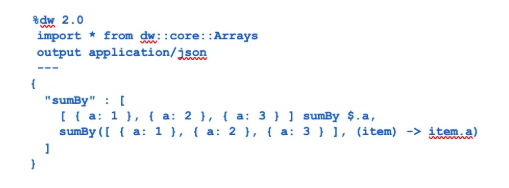Output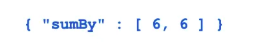1. take

Selects first n elements. It returns an empty array when n <= 0 and the original array when n > sizeOf(array).

Parameters

• The left Array of elements.
• The number of elements to take.

Example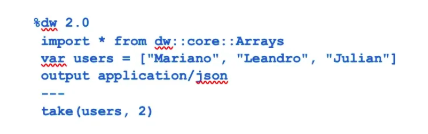Output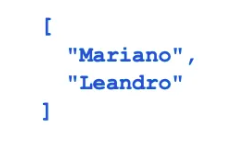1. takeWhile

Takes elements for the array while the condition is met.

Parameters

• The Array of elements.
• The condition (or expression) used to match an element in the array.

Example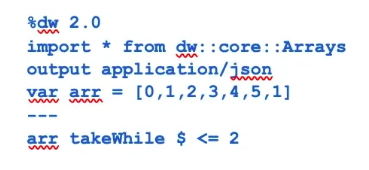Output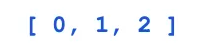Topics:
integration ,tutorial ,arrays module in dataweave ,power of arrays

Comment (0)

Save
{{ articles.views | formatCount}} Views

Published at DZone with permission of Lalit Panwar . See the original article here.

Opinions expressed by DZone contributors are their own.